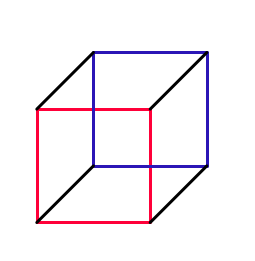Think 2-D Is Cool? Wait Till You Try 3-D!A lattice cube is a cube in 3-dimensional space, whose vertices are lattice points. Describe the set of possible side lengths of a lattice cube.

Note: A lattice point is a point whose coordinates are all integers. $(0,0,1)$ is a lattice point, but $(\frac{1}{2}, \sqrt{2}, -0.2 )$ is not a lattice point.

For similar problems, you can read my note on Construction.

×